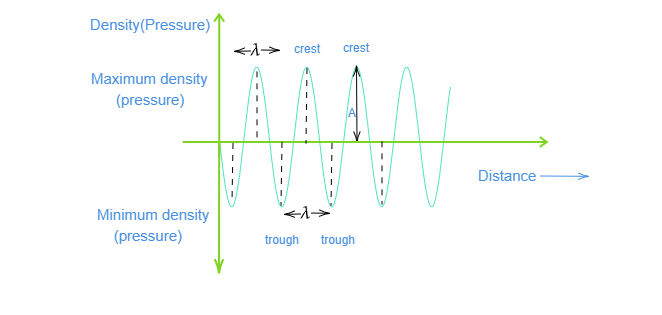# Draw a curve showing density or pressure variations with respect to distance for a disturbance produced by sound. Mark the position of compression and rarefaction on this curve. Also define wavelengths and time period using this curve.Crests and troughs: are the points of maximum and minimum density respectively.

Time period$(T)$: In the given graph, time taken to cross its two consecutive crests or troughs is called time period of the wave.

Wavelength$(\lambda)$: In the given graph, distance between two consecutive crests or troughs is the wavelength of the wave.